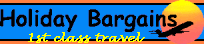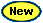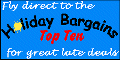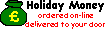<% Dim strCategory, strArea, strUnit, strDuration, strPrice, strNotes Dim Conx, RecSet strCategory=request.querystring("Category") strArea=request.querystring("Area") strUnit=request.querystring("Unit") strDuration=request.querystring("Duration") Set Conx = server.createobject("adodb.connection") Conx.Provider = "Microsoft.Jet.OLEDB.4.0" Conx.Open server.mappath("kh_data.mdb"), "admin", "" Set RecSet = server.createobject("adodb.recordset") Select Case strCategory Case "1" 'General RecSet.Open "SELECT Top 1 Duration, Price FROM tb_GenInsurance WHERE AreaID = " & strArea & " AND UnitID = " & strUnit & " AND Duration >= " & strDuration & " ORDER BY Duration", Conx, , , adCmdText strDuration=RecSet("Duration") Case "2" 'Ski if strArea = "3" then strArea = "4" if strUnit = "5" then strUnit = "4" RecSet.Open "SELECT Top 1 Duration, Price FROM tb_SkiInsurance WHERE AreaID = " & strArea & " AND UnitID = " & strUnit & " AND Duration >= " & strDuration& " ORDER BY Duration", Conx, , , adCmdText strDuration=RecSet("Duration") Case "3" 'General if strUnit = "3" then strUnit = "1" RecSet.Open "SELECT Price FROM tb_AnnInsurance WHERE UnitID = " & strUnit, Conx, , , adCmdText End Select strPrice=RecSet("Price") RecSet.Close RecSet.Open "SELECT Notes FROM tb_InsuranceNotes WHERE CategoryID = " & strCategory & " AND UnitID = " & strUnit, Conx, , , adCmdText strNotes=RecSet("Notes") RecSet.Close Conx.Close Set RecSet = Nothing Set Conx = Nothing %> Travel Insurance from Holiday BargainsTravel InsuranceTravel InsuranceCar Hire Pricebusters Spain from £59pw
discounted rates from Holiday Bargains
Our travel insurance can be half the price of some High Street Travel Agents... and you don't have to book your holiday with us to get these discounted rates (although it might be wise to do so)!

Enter your insurance requirements below and click the 'Price' button to get a quote. To book, just give us a call on 08707 571 571 and we'll have you covered in minutes.Category <%If strCategory = "1" then%> <%else%> <%end if%> General single trip Holiday Bargains Price <%If strCategory = "2" then%> <%else%> <%end if%> Ski single trip <%=strPrice%> <%If strCategory = "3" then%> <%else%> <%end if%> Annual Area <%If strCategory = "3" then%> Worldwide <%else%> <%If strArea = "1" then%> UK <%else%> UK <%End If%> <%If strArea = "2" then%> Europe <%else%> Europe <%End If%> <%If strArea = "3" then%> Australia & New Zealand <%elseif strCategory <> "2" Then%> Australia & New Zealand <%End If%> <%If strArea = "4" then%> Worldwide <%else%> Worldwide <%End If%> <%End If%> Notes <%=strNotes%> Cover Type <%If strUnit = "1" then%> Adult <%else%> Adult <%End If%> <%If strUnit = "3" then%> Child <%elseif strCategory <> "3" Then %> Child <%End If%> <%If strUnit = "4" then%> Family <%else%> Family <%End If%> <%If strUnit = "5" then%> Single parent family <%elseif strCategory <> "2" Then%> Single parent family <%End If%> Duration <%if strCategory = "3" Then%> xxxxxxxxxxxxxxx <%else%> <%if strCategory = "1" Then%> <%If strDuration = "1" then%> 1 day <%else%> 1 day <%end if%> <%If strDuration = "3" then%> up to 3 days <%else%> up to 3 days <%end if%> <%If strDuration = "4" then%> up to 4 days <%else%> up to 4 days <%end if%> <%If strDuration = "9" then%> up to 9 days <%else%> up to 9 days <%end if%> <%else%> <%If strDuration = "5" then%> up to 5 days <%else%> up to 5 days <%end if%> <%If strDuration = "8" then%> up to 8 days <%else%> up to 8 days <%end if%> <%If strDuration = "10" then%> up to 10 days <%else%> up to 10 days <%end if%> <%End If%> <%If strDuration = "12" then%> up to 12 days <%else%> up to 12 days <%End If%> <%If strDuration = "18" then%> up to 18 days <%else%> up to 18 days <%End If%> <%If strDuration = "25" then%> up to 25 days <%else%> up to 25 days <%End If%> <%If strDuration = "32" then%> up to 32 days <%else%> up to 32 days <%End If%> <%End If%> 08707 571 571   Summary of Cover   Summary of Conditions   Email usHome | Top Ten Bargains | The Boards | Enquiry Form | Quotebuster | Market Place | Mailing Lists | Resource Centre | Travel IndustryAbout us | Contact us | Link to us                      Site Map | Settings